Question

(a) Let P(B1∩B2)>0, and A1∪A2⊂B1∩B2. Then show that P(A1|B1).P(A2|B2)=P(A1|B2).P(A2|B1). (b) Let A and B1 be independent;...

(a) Let P(B1∩B2)>0, and A1∪A2⊂B1∩B2. Then show that

P(A1|B1).P(A2|B2)=P(A1|B2).P(A2|B1).

(b) Let A and B1 be independent; similarly, let A and B2 be independent. Show that in this case, A and B1∪B2 are independent if and only if A and B1∩B2 are independent.

(c) Given P(A) = 0.42,P(B) = 0.25, and P(A∩B) = 0.17, find

(i)P(A∪B) ;

(ii)P(A∩Bc) ;

(iii)P(Ac∩Bc) ;

(iv)P(Ac|Bc).

Complete solution is given in attached images: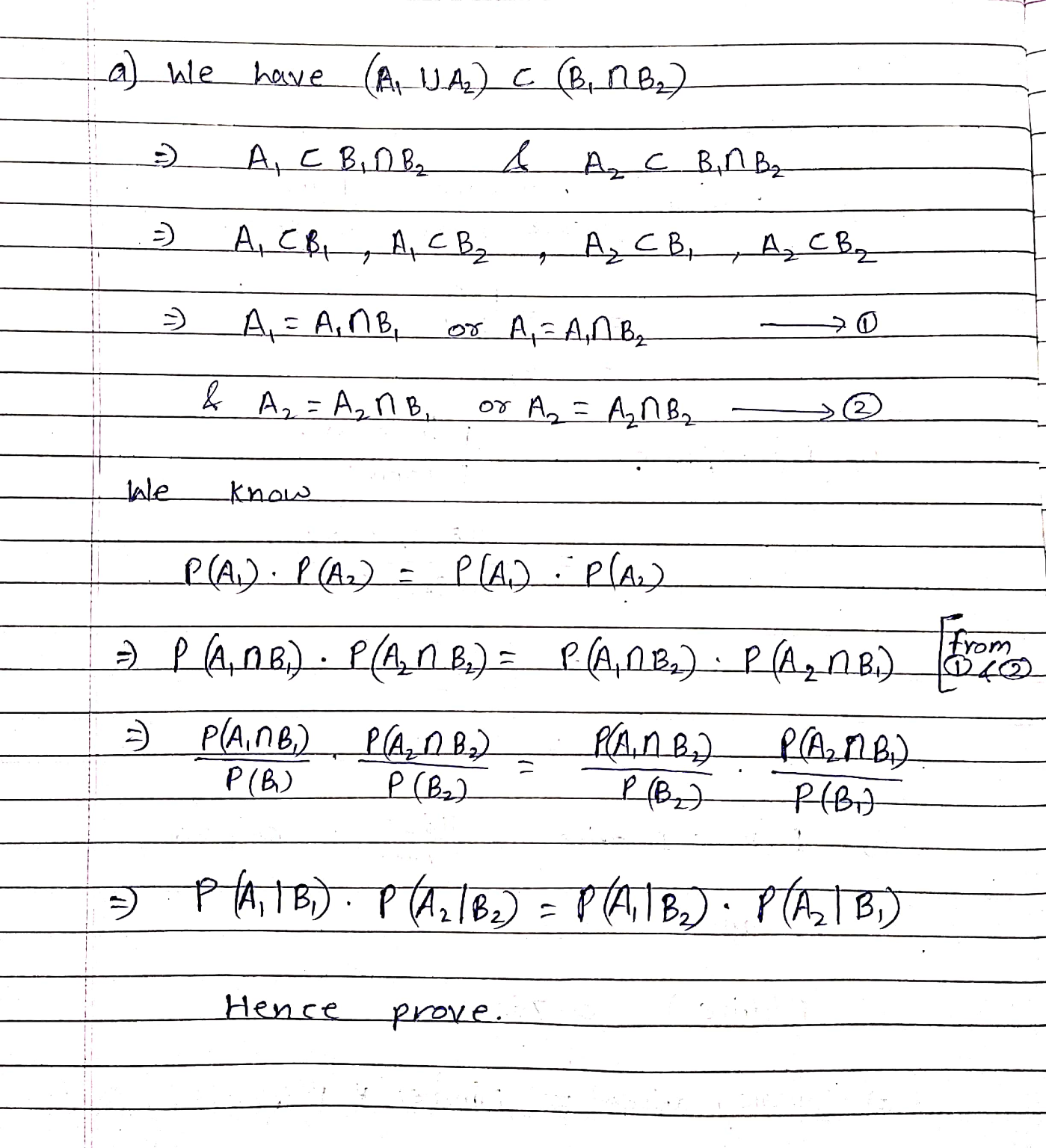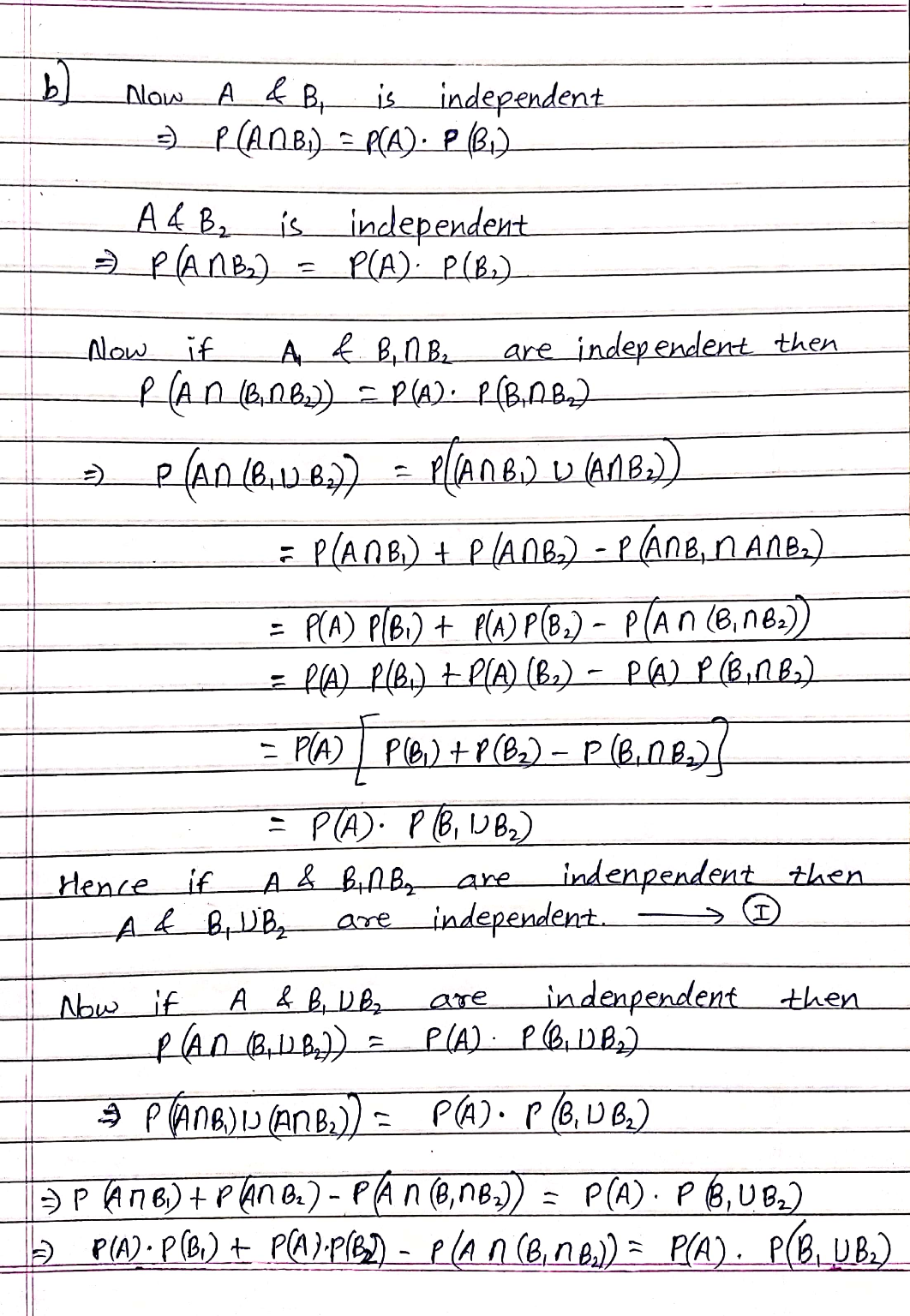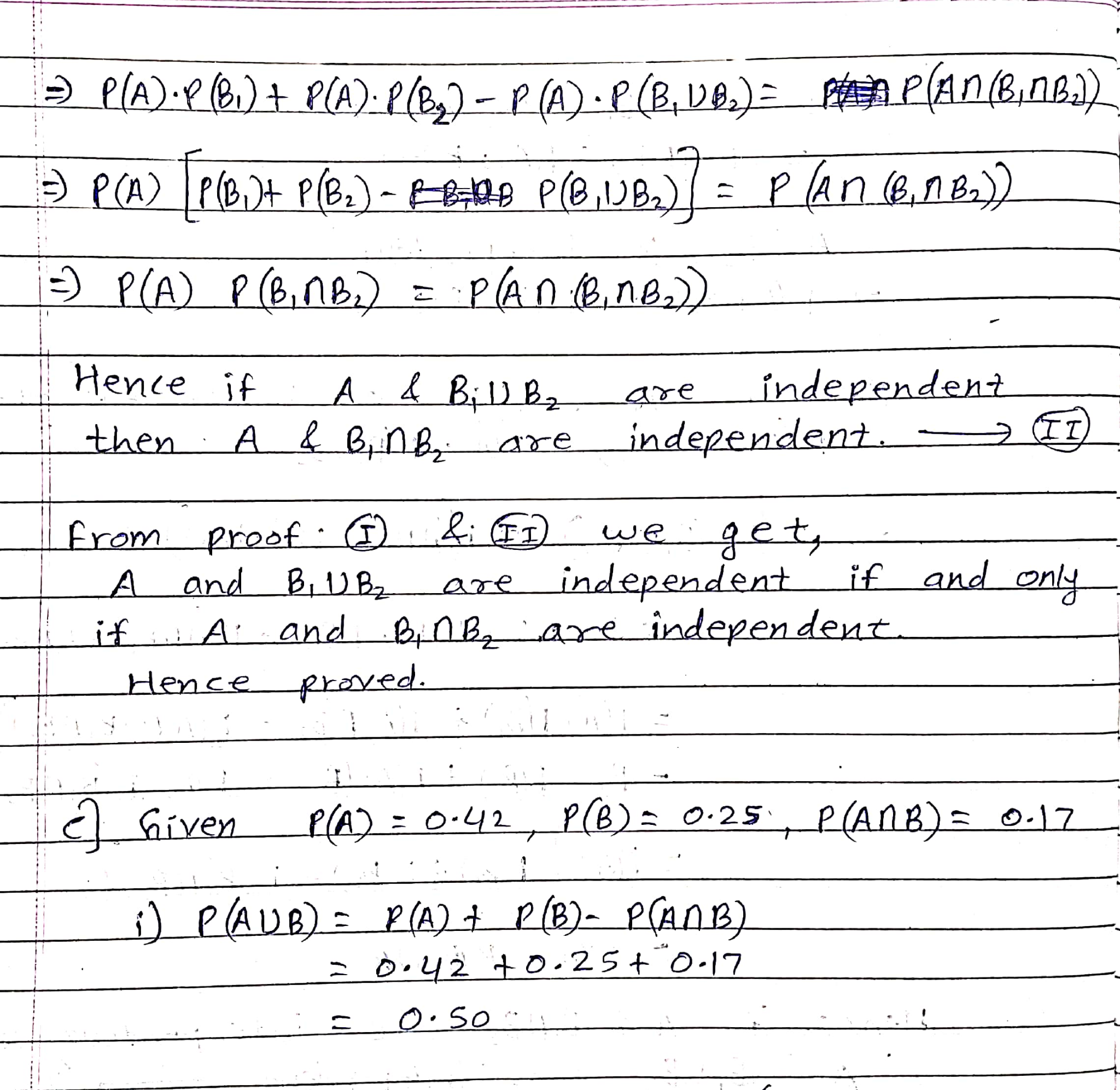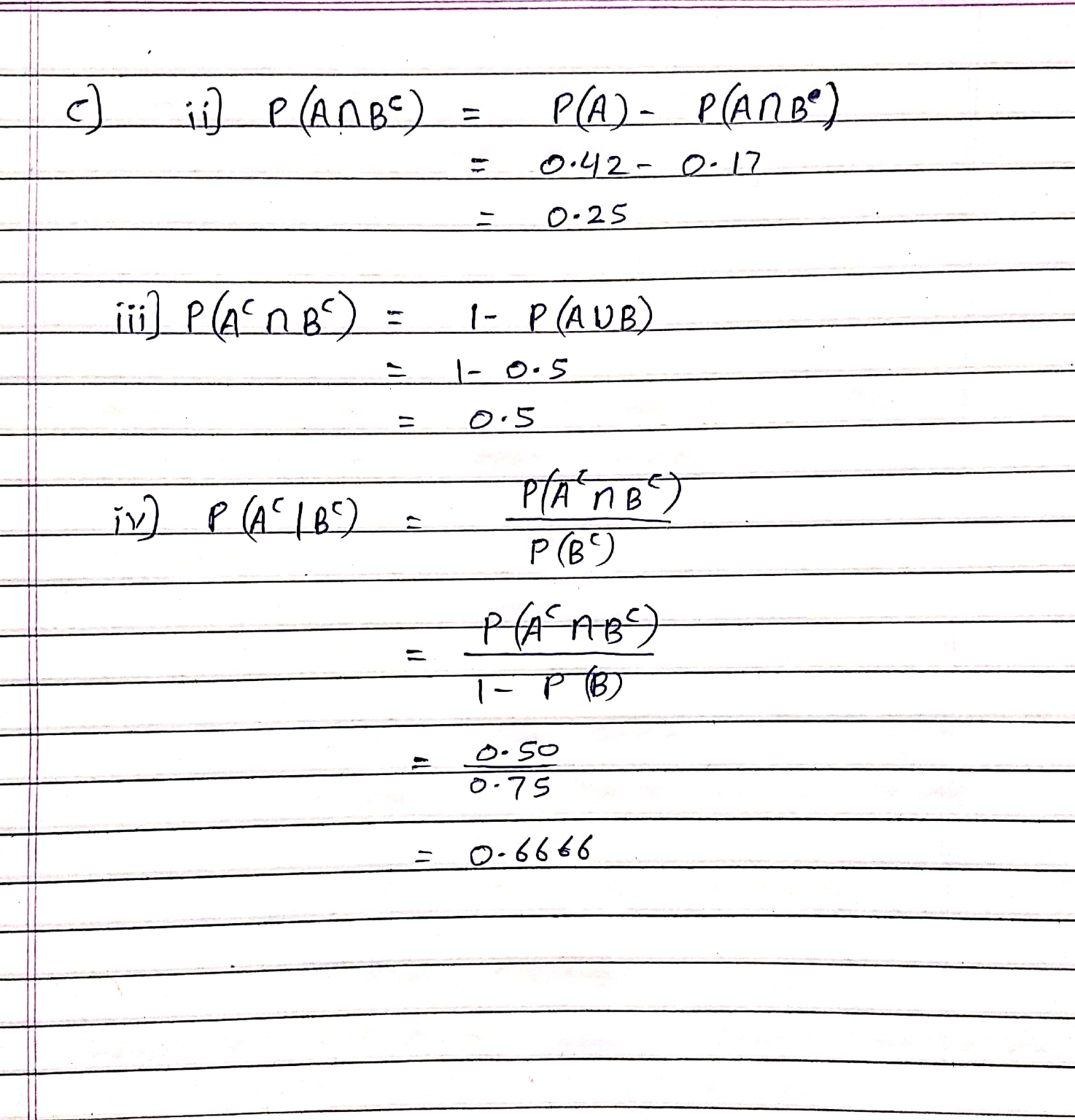Thank You.

Earn Coins

Coins can be redeemed for fabulous gifts.

Similar Homework Help Questions
• 2. (a) Let P(Bin B2) > 0, and AUA, CBin B2. Then show that P(A/B).P (A2|B2)...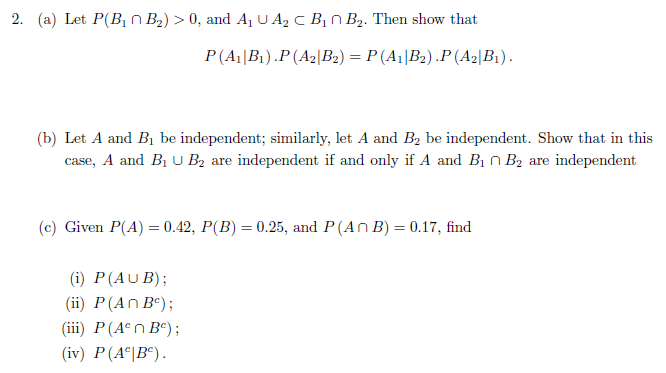2. (a) Let P(Bin B2) > 0, and AUA, CBin B2. Then show that P(A/B).P (A2|B2) = P(A|B2).P (A2|Bi). (b) Let A and Bbe independent; similarly, let A and B, be independent. Show that in this case, A and B U B2 are independent if and only if A and Bin B2 are independent (c) Given P(A) = 0.42, P(B) = 0.25, and P(An B) = 0.17, find (i) P (AUB); (ii) P(An B°); (iii) P(A n B); (iv) P(...

• Show that the formula for a line through two points (a1,b1) and (a2,b2) is: y=(b1-b2)/(a1-a2) * x + (a1*b2-a2*b1)/(a1-a2) The slope part looks right

Show that the formula for a line through two points (a1,b1) and (a2,b2) is: y=(b1-b2)/(a1-a2) * x + (a1*b2-a2*b1)/(a1-a2) The slope part looks right. Could someone explain how the y-intercept part makes sense? I would think that the y-intercept is: b1-slope*a1 or b2-slope*a2 anyone have any ideas?

• GAME 3 Player B B1 B2 Player A A1 7,3 5, 10 A2 3,8 9,6 In...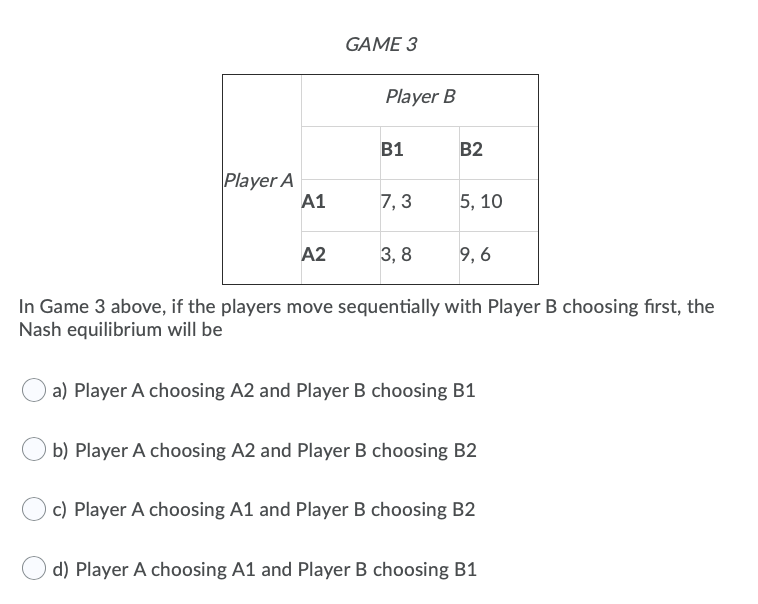GAME 3 Player B B1 B2 Player A A1 7,3 5, 10 A2 3,8 9,6 In Game 3 above, if the players move sequentially with Player B choosing first, the Nash equilibrium will be a) Player A choosing A2 and Player B choosing B1 b) Player A choosing A2 and Player B choosing B2 c) Player A choosing A1 and Player B choosing B2 d) Player A choosing A1 and Player B choosing B1

• Given these probabilities, complete the contingency table, and compute the following probabilities: a) P(A2 and B1)...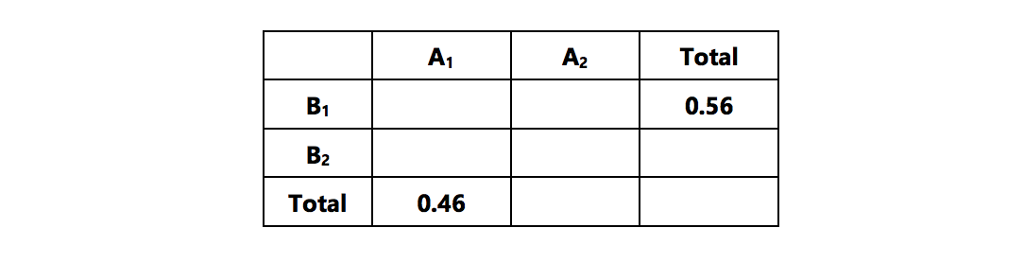Given these probabilities, complete the contingency table, and compute the following probabilities: a) P(A2 and B1) b) P(A1 | B1) c) P(B2 | A2) d) P(B2 or A1) A1 A2 Total B1 0.56 B2 Total 0.46

• given the following joint probability table A1 A2 B1 .02 .01 B2 .05 .02 Calculate the...

given the following joint probability table A1 A2 B1 .02 .01 B2 .05 .02 Calculate the conditional probability P(A1IB1) round your answer

• float useless(A){ n = A.length; if (n==1) { return A[@]; let A1,A2 be arrays of size...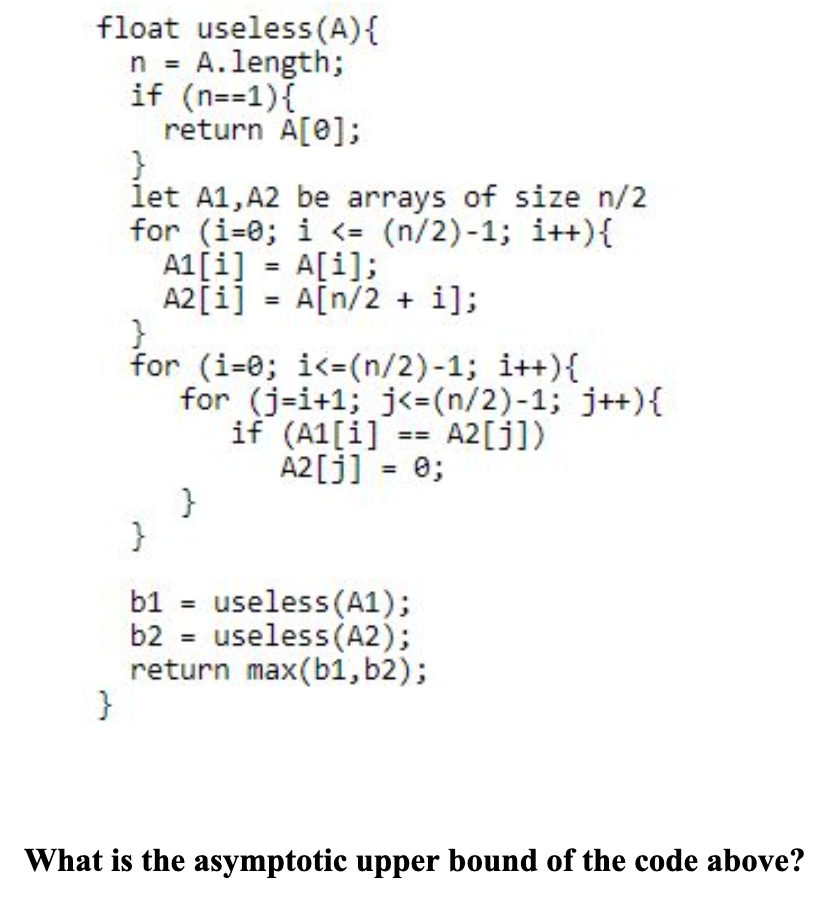float useless(A){ n = A.length; if (n==1) { return A[@]; let A1,A2 be arrays of size n/2 for (i=0; i <= (n/2)-1; i++){ A1[i] = A[i]; A2[i] = A[n/2 + i]; for (i=0; i<=(n/2)-1; i++){ for (j=i+1; j<= (n/2)-1; j++){ if (A1[i] == A2[j]) A2[j] = 0; b1 = useless(A1); b2 = useless (A2); return max(b1,b2); What is the asymptotic upper bound of the code above?

• (14) Show that if [(a1,b)- [(a2, b2) and [c,d) (c2, d2)] and bidi(aidi -b)>0 then b2d2(azd2 - b2c...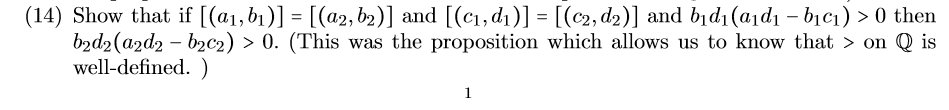(14) Show that if [(a1,b)- [(a2, b2) and [c,d) (c2, d2)] and bidi(aidi -b)>0 then b2d2(azd2 - b2c2) > 0. (This was the proposition which allows us to know that > on Q is well-defined. ) (14) Show that if [(a1,b)- [(a2, b2) and [c,d) (c2, d2)] and bidi(aidi -b)>0 then b2d2(azd2 - b2c2) > 0. (This was the proposition which allows us to know that > on Q is well-defined. )

• Urgent!! Please show mark all correct answers and also find values of a1,a2,a3,a4,a5,a6 and b1,b2,b3,b4,b5,b6. Thank...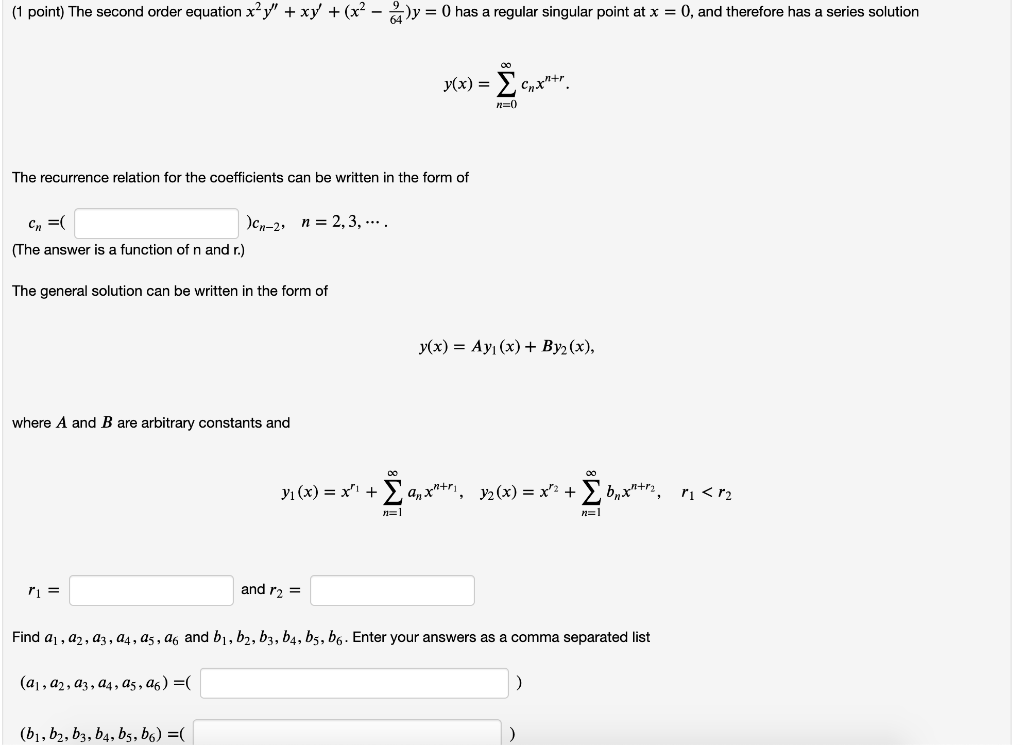Urgent!! Please show mark all correct answers and also find values of a1,a2,a3,a4,a5,a6 and b1,b2,b3,b4,b5,b6. Thank you! (1 point) The second order equation x?y" + xy' +(x2 - y = 0 has a regular singular point at x = 0, and therefore has a series solution y(x) = Σ CGxhtr P=0 The recurrence relation for the coefficients can be written in the form of n = 2, 3, ... C =( Jan-2 (The answer is a function of n and...

• 1. Prove“inclusion-exclusion,”thatP(A∪B)=P(A)+P(B)−P(A∩B). 2. Prove the “unionbound, ”thatP(A1∪A2)≤P(A1)+P(A2). Under what conditions does the equality hold? 3. Provethat,...

1. Prove“inclusion-exclusion,”thatP(A∪B)=P(A)+P(B)−P(A∩B). 2. Prove the “unionbound, ”thatP(A1∪A2)≤P(A1)+P(A2). Under what conditions does the equality hold? 3. Provethat, for A1 andA2 disjoint, P(A1∪A2|B)=P(A1|B)+P(A2|B). 4. A and B are independent events with nonzero probability. Prove whether or not A and Bc are independent.

• 6. Consider two possible networks for connecting points X and Y: a1 bi Л. C) b2...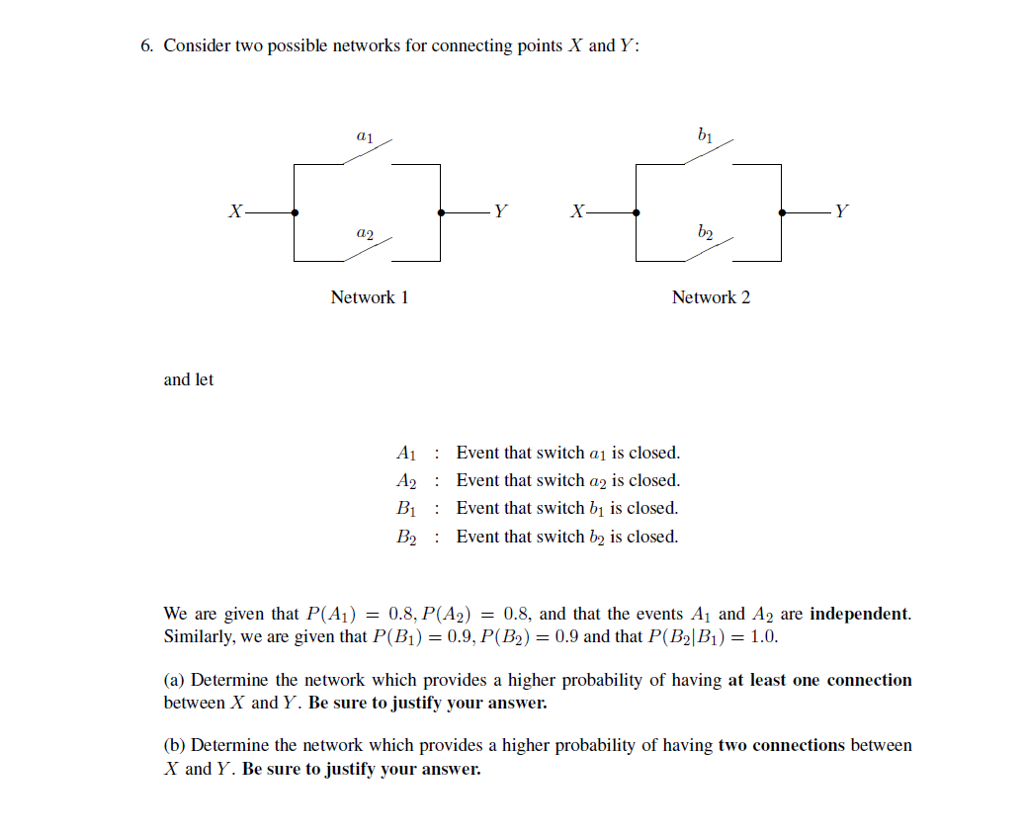6. Consider two possible networks for connecting points X and Y: a1 bi Л. C) b2 a2 Network 1 Network 2 and let A : Event that switch ai įs closed. A2Event that switch a2 is closed. Bi : Event that switch bi s closed B2 Event that switch b is closed We are given that P(A) = 0.8,P(A2) = 0.8, and that the events A1 and A2 are independent. Similarly, we are given that P(B) = 0.9. P(B) =...